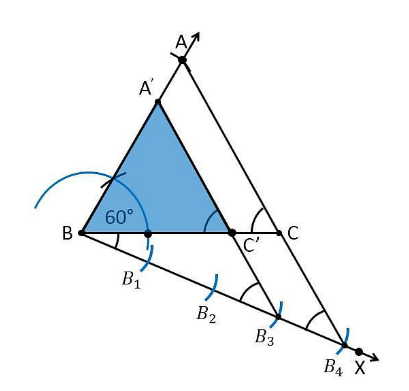Guru

# Draw a triangle ABC with side BC = 6 cm, AB = 5 cm and ∠ABC = 60°. Then construct a triangle whose sides are 3/4 of the corresponding sides of the triangle ABC. Q.5

• 0

What is the best way to solve the problem of class 10th ncert of construction chapter of exercise 11.1 of question no.5, find the best way to solve this problem Draw a triangle ABC with side BC = 6 cm, AB = 5 cm and ∠ABC = 60°. Then construct a triangle whose sides are 3/4 of the corresponding sides of the triangle ABC.

Share

1. 1. Draw a ΔABC with base side BC = 6 cm, and AB = 5 cm and ∠ABC = 60°.

2. Draw a ray BX which makes an acute angle with BC on the opposite side of vertex A.

3. Locate 4 points (as 4 is greater in 3 and 4), such as B1, B2, B3, B4, on line segment BX.

4. Join the points B4C and also draw a line through B3, parallel to B4C intersecting the line segment BC at C’.

5. Draw a line through C’ parallel to the line AC which intersects the line AB at A’.

6. Therefore, ΔA’BC’ is the required triangle.Justification:

The construction of the given problem can be justified by proving that

Since the scale factor is 3/4 , we need to prove

A’B = (3/4)AB

BC’ = (3/4)BC

A’C’= (3/4)AC

From the construction, we get A’C’ || AC

In ΔA’BC’ and ΔABC,

∴ ∠ A’C’B = ∠ACB (Corresponding angles)

∠B = ∠B (common)

∴ ΔA’BC’ ∼ ΔABC (From AA similarity criterion)

Since the corresponding sides of the similar triangle are in the same ratio, it becomes

Therefore, A’B/AB = BC’/BC= A’C’/AC

So, it becomes A’B/AB = BC’/BC= A’C’/AC = 3/4

Hence, justified.

• 0